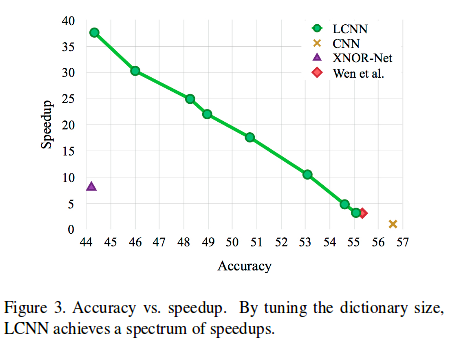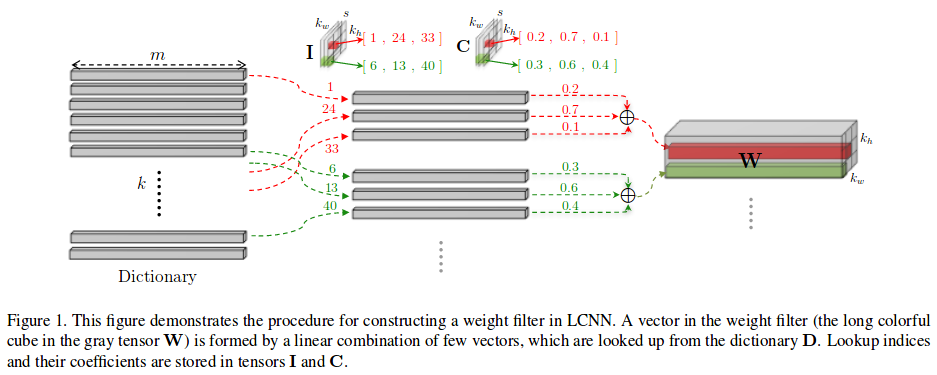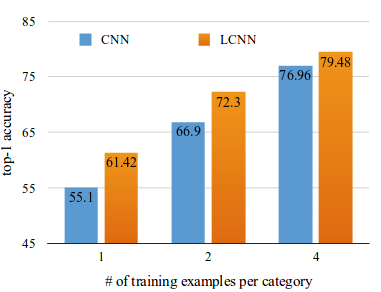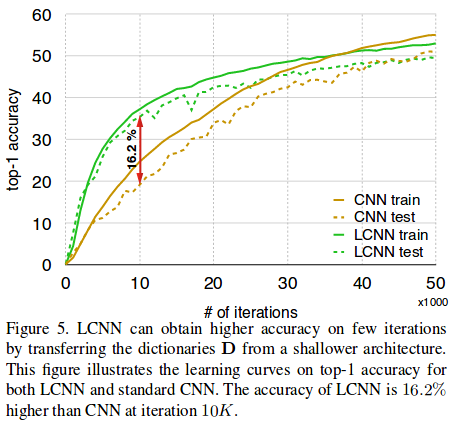LCNN is a novel layer that can replace any convolutional layer. The main thing is that a filter is “decomposed” as a linear combination of parts from a dictionary. The ability to tune the dictionary size and the number of elements in the linear combination allows an efficiency/accuracy trade-off (see Figure 3). In addition, the authors argue that LCNN has an advantage in few-shot learning and few-iteration learning settings.# Filter Construction

Let’s first define the 4 tensors that we’ll need:

• $$\bold{W} \in \mathbb{R}^{m \times k_w \times k_h}$$ is a filter, where $$(k_w, k_h)$$ are its spatial dimensions and $$m$$ is the number of channels in the input image.
• $$\bold{D} \in \mathbb{R}^{k \times m}$$ is the dictionary. It contains $$k$$ vectors of size $$m$$. Those vectors will be combined to construct the filter (See Figure 1).
• $$\bold{I} \in \mathbb{N}^{s \times k_w \times k_h}$$ is the lookup indices tensor, where $$s$$ is the number of elements in the linear combination.
• $$\bold{C} \in \mathbb{R}^{s \times k_w \times k_h}$$ is the coefficient tensor.

Then, we can formulate the construction as follows:

$\bold{W}_{[:,r,c]} = \sum_{t=1}^s \bold{C}_{[t,r,c]} \cdot \bold{D}_{[\bold{I}_{[t,r,c]},:]}$# Fast Convolution using a Shared Dictionary

Now, let’s imagine that our dictionary is in fact the weights of a 1x1 convolution layer: we thus have $$k$$ filters of dimensions $$(m, 1, 1)$$. By passing our input image $$\bold{X} \in \mathbb{R}^{m \times w \times h}$$ through this 1x1 convolutional layer, we get $$\bold{S} \in \mathbb{R}^{k \times w \times h}$$. With $$\bold{I}$$ and $$\bold{C}$$ in hand, we can compute the result of the convolution $$\bold{X} * \bold{W}$$ for each filter, without really constructing any $$\bold{W}$$. (See section 3.1.1 of paper.) By reducing $$k$$ and $$s$$, we can reduce the number of lookups and floating point operations.

One problem arises from using indices to select values in tensors: it makes the network non-differentiable. During training, a trick is used to be able to backpropagate. For each filter $$\bold{W}$$, a sparse tensor $$\bold{P} \in \mathbb{R}^{k \times k_w \times k_h}$$ is constructed. In this tensor, elements at indices $$\bold{I}$$ have values $$\bold{C}$$. Then, we have that $$\bold{X} * \bold{W} = \bold{S} * \bold{P}$$ for each $$\bold{W}, \bold{P}$$ pair.

During training, we learn $$\bold{D}$$ and $$\bold{P}$$. To obtain a tensor $$\bold{P}$$ that is $$s$$-sparse, we impose a $$\ell_1$$ norm and keep only $$s$$ non-zero elements. From $$\bold{P}$$, we can easily obtain $$\bold{I}$$ and $$\bold{C}$$.

# Few-shot learning

The authors argue that this layer architecture has a performance advantage in few-shot learning settings, as we can see in the following plot (extracted from Figure 4).# Few-iteration learning

The authors also argue that LCNN can learn more in the first iterations of training than an ordinary CNN. In one experiment, they transferred the dictionary learned with a shallow network to a deeper network and only trained $$\bold{I}$$ and $$\bold{C}$$. See Figure 5 for the learning curves.